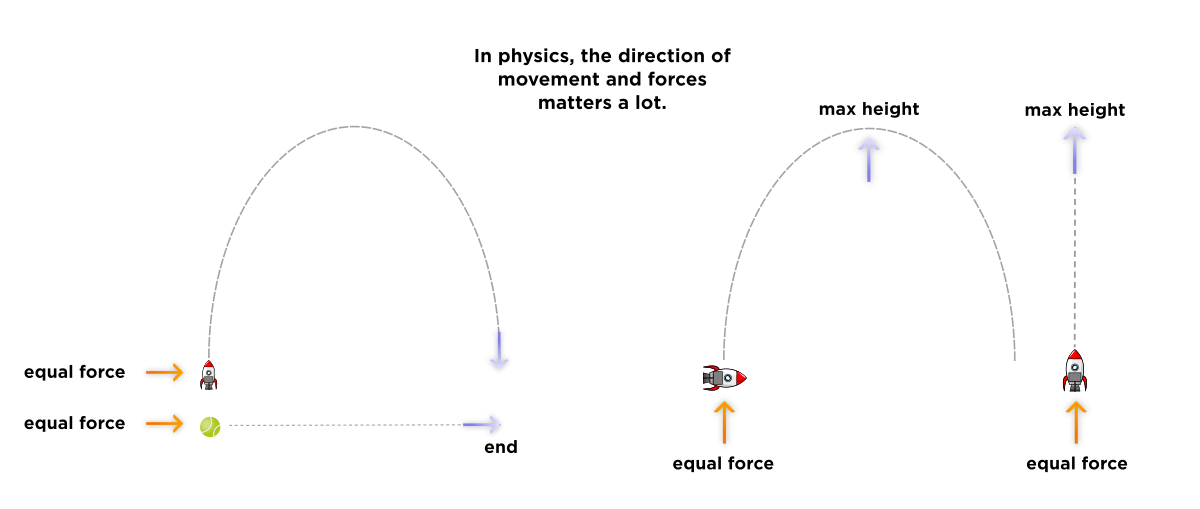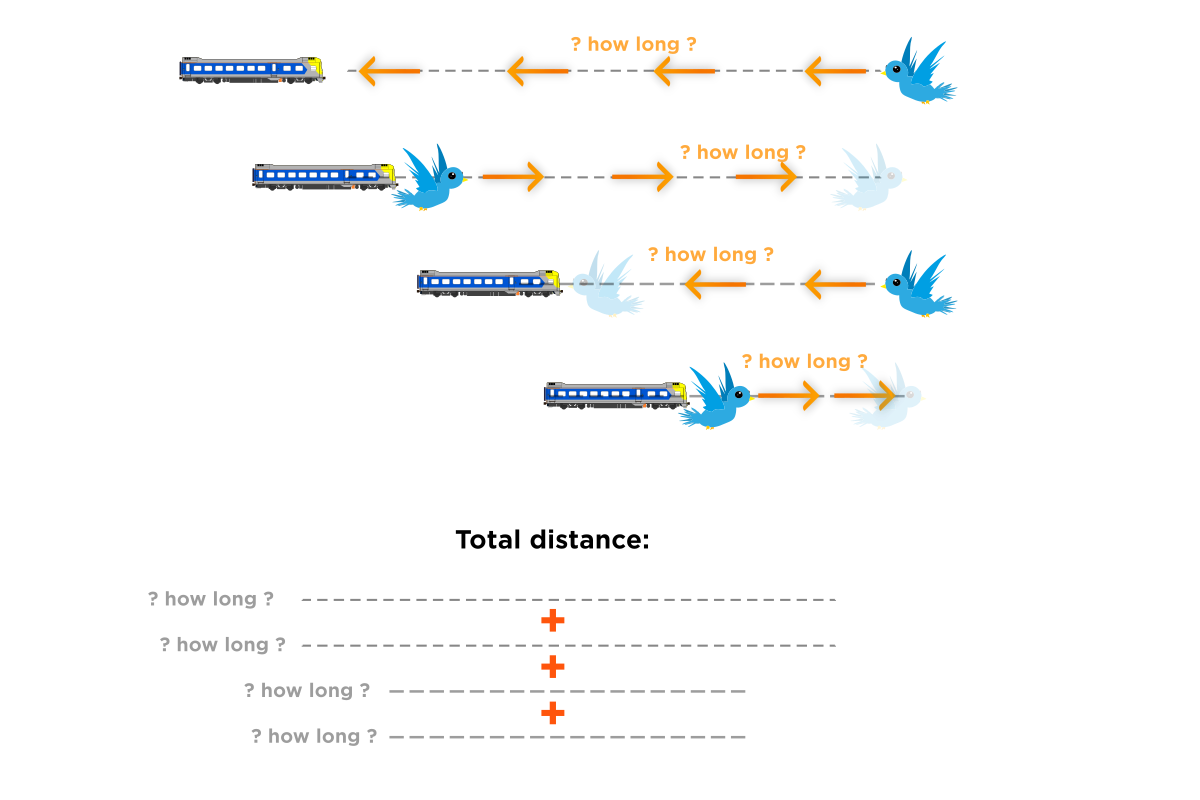# Question 19

• Problem:
Train stations A and B are connected by a straight track that is 72 miles long. A slow freight train traveling at 18 miles per hour leaves A and heads towards B. At the same time, a bird who flies at 30 miles per hour leaves B and heads towards A. When the bird meets the train, it turns around "instantaneously" (that is, imagine it gets reflected) and heads back towards B. When the bird reaches the station, it turns around and heads back towards A until it meets the train. Then bird then turns around again and heads back towards the station. This process continues until the train reaches B. What is the total distance flown by the bird?

Explanation:
We can essentially ignore the bird turning around and imagine that it instead flies continuously straight. It takes the train 72/18=4 hours to reach the opposite station, and during this time, the bird will fly 30 * 4 = 120 miles.

Why can you assume that the bird doesn't turn around?

• It's a simulation almost. Read the explanation carefully. It's proposing a new perspective to look at the problem with.

• This is a really good question!

Physics teaches us to learn to always taking direction into consideration when doing calculations. Instead of speed, we use the quantity velocity, which you can think of as an arrow with length equal to the speed, pointing in the direction of the object's motion.

A force applied to an object only affects the motion of the object which is in the same direction. In the diagram below, you can see on the left that the rocket and the ball both have a horizontal force acting on them, but since the rocket has a vertical thrust upwards, it moves in a parabolic curve. Importantly, they go the same horizontal distance, since the horizontal forces acting on them are the same, so they end at the same spot!

On the right, we see that a vertical force applied upwards (like if we were kicking the rockets upwards) causes both rockets to reach the same height, and this height isn't affected by the horizontal thrust that the left-side rocket has by itself.The simplest way to analyze physics questions using direction is to describe a vector as the aggregate (the "sum") of the $$x$$ and $$y$$ "parts" of the velocity, if our space is 2-dimensional, as with the diagrams above. The way to do this is to draw a right triangle with the velocity vector as the hypotenuse. The $$x$$ and $$y$$ parts of the velocity are simply the horizontal and vertical legs of this right triangle. We can use trigonometric functions like $$\cos \theta$$ and $$\sin \theta$$ Pythagorean Theorem to figure out the lengths of the other two sides.

### What does this have to do with the question about the bird flying?

Well, the natural thing to do with this question is to map out the bird's flight as a zig-zag path going both directions right and left.After all, in physics, we carefully bookmark each vector direction of our velocity, force, and momentum. So the trick used in the question, to "flatten out" the bird's flight by un-zig-zagging it, is a bit counter-intuitive. This is what is meant by "ignoring the bird turning around." It is to regard the tunnel zig-zag bird's flight as being the same length as that of a bird that does not turn around.

## The trick is to not do the above, which is to add the segments of the bird's journey

The trick is to pretend that the bird flies in a straight line. This makes it easier to visualize how to do this problem, as we see a simple straight path with constant speed that we can apply the rate equation to!

(To be continued!)

• This post is deleted!

• Here's another way to write the solution.
The bird is traveling at 30 miles per hour no matter what. The bird never slows down or speeds up. Because of this, the bird must be traveling at a constant speed. Since it's traveling at a constant speed, we can just find the distance by multiplying 30 by the time, which is 72/18=4. The answer is 120.